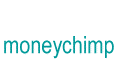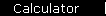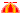# Summing a Geometric Series

So far we've been looking at "one time" investments, like making a single deposit to a bank account. But many finance problems involve other periodic adjustments to your balance, like a savings account or a mortgage where you make regular contributions, or an annuity where you make regular withdrawals. The formulas for these "periodic" effects are based on finding the sum of a geometric series.

So suppose z is some number you are interested in, and lets say you want to add all the powers of z up to the 30th power:

 1 1 + z + z2 + z3 + . . . + z30

How are you going to calculate this monster sum?

Well, one way you could do it is to get some sharp pencils and a hell of a lot of paper and start grinding through 60 separate sums and products; and you'll want to do it twice, just to make sure you didn't mess something up the first time. Naturally, you'll pass on this method and search for a better plan.

So let's try a scientific approach. We'll define a function f( ), and let f(n) equal the sum of the first n terms of our series:

 2 f(n) = 1 + z + z2 + z3 + . . . + zn

The goal here is to get some simple formula for f(n).

There is no way to grind through this to find the answer. You need to use a trick. The trick is to ask yourself this question: Suppose I know the formula for f(n); how do I find f(n+1)?

The answer to that is obvious -- you just add on the next power of z:

 3 f(n + 1) = f(n) + zn+1

However, there's a second way you could get f(n + 1): take the terms in f(n), multiply them all by z to bump the powers up, and then add 1 in front of the whole thing:

 4 f(n + 1) = 1 + z . f(n)

Now take a look at equations 3 and 4: they both contain f(n), which is what we want. The left sides of the two equations are equal, so we set the right sides equal, and solve algebraically for f(n):

 1 + z . f(n) = f(n) + zn+1 z . f(n) - f(n) = zn+1 - 1 f(n)[z - 1] = zn+1 - 1 5. f(n) = [zn+1 - 1]/[z - 1]

So we put equations 2 and 5 together to get what we're looking for:

 1 + z + z2 + z3 + . . . + zn   =   [zn+1 - 1]/[z - 1]

Sometimes, we'll want to do a series without that leading "1". To get a formula for that, you can either go through the same reasoning we used above (that's a fun exercise), or you can just subtract 1 from both sides and simplify the fraction. We'll just write down the result:

 z + z2 + z3 + . . . + zn   =   [zn+1 - z]/[z - 1]One interesting special case is the infinite series, meaning that you add up

 z + z2 + z3 + . . . + zn + . . .

and just keep going, forever. It sounds like a crazy idea, but it's really used, for example, in the theory of stock prices. The price you're willing to pay for a share of stock depends on what you think someone else will pay sometime in the future when you're ready to sell; and his price depends on what he thinks somebody else will pay even further in the future, and so on, forever. So the price you pay for stock right now reflects people's expectations about the company's earnings from now to eternity.

The infinite series will converge to a finite result as long as z is between 0 and 1, and the formula is simple: just take [zn+1 - z]/[z - 1], and notice that zn+1 goes to zero as n goes to infinity. So you get

 z + z2 + z3 + . . . + zn + . . .   =   [ - z]/[z - 1] (for 0 <= z < 1)

home  |  article  |  glossary  |  calculator  |  about us  |  booksIntroductionCompound InterestMore CompoundingPresent Value / CAGRComposite InvestmentsBond YieldGeometric SeriesGrowth + ContributionsAnnuityMortgageStock ValuationBooks & Links